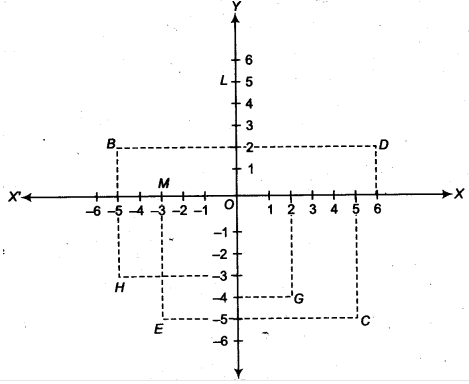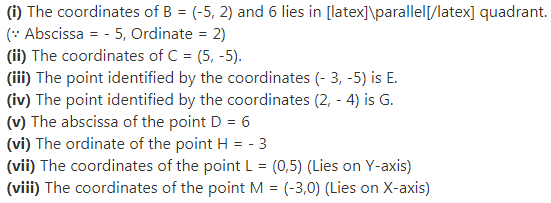# See figure, and write the following

See figure, and write the following(i) The coordinates of B.
(ii) The coordinates of C.
(iii) The point identified by the coordinates (-3, - 5).
(iv) The point identified by the coordinates (2, -4).
(v) The abscissa of the point D.
(vi) The ordinate of the point H.
(vii) The coordinates of the point I.
(viii) The coordinates of the point M.